< Back to Listing

## Cycle Counting by the Probabilities

Why the best systems weigh the inventory-variance odds
September/October 2016

The most effective cycle counting programs reflect the realities of managing inventory in a dynamic environment. Within successful frameworks such as these, inventory managers are able to reach their inventory accuracy goals and balance the cost of cycle counting with the cost of analyzing variance causes and maintaining accuracy controls. Unfortunately, many programs suffer from ambiguity and imprecision related to the mechanics of assembling the cycle counting system. Furthermore, too many inventory managers struggle to determine the optimum cycle for item review, how many items to count, and how to use cycle counting results to continuously improve record accuracy.

Perhaps the best way to begin addressing these problems is to define cycle counting. According to the APICS Dictionary, 14th edition, cycle counting is: “An inventory accuracy audit technique where inventory is counted on a cyclic schedule rather than once a year. A cycle inventory count is usually taken on a regular, defined basis (often more frequently for high-value or fast-moving items and less frequently for low-value or slow-moving items). Most effective cycle counting systems require the counting of a certain number of items every workday with each item counted at a prescribed frequency. The key purpose of cycle counting is to identify items in error, thus triggering research, identification, and elimination of the cause of the errors.”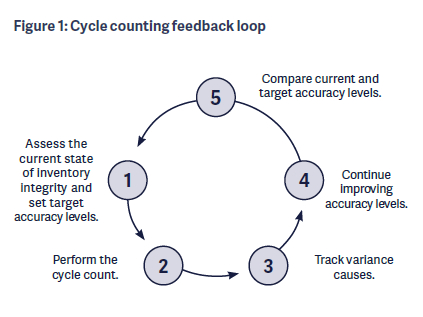As illustrated in Figure 1, the cycle counting function should be understood as a closed-loop process composed of the following five steps:

1. Assess the current state of inventory integrity and set target accuracy levels. The opening step in the cycle counting process is to determine the accuracy level of the current inventory. Inventory managers should validate key inventory control elements such as stocking locations; locator systems; procedures for inventory receiving, put away, and picking; and transaction management. Once the parameters of inventory integrity are established, inventory control can determine the current level of accuracy. Then, a realistic target level is set. Also, inventory control should establish what cycle counting method is to be used, how many items are counted each day, their recurring cycle, what procedures govern how the count is to be performed, and the teams involved.
2. Perform the cycle count. The cycle counting process begins. A select number of items is counted each day.
3. Track variance causes. The results of the cycle count are submitted to the inventory-variance tracking team. These people are responsible for assembling the results, comparing physical counts with book balances, identifying which counts are accept-able and unacceptable, investigating and assigning reason codes for variances, performing reconciliation transaction updates, working with warehouse managers to determine the root causes of variances, selecting items for recounting, and preparing the next day’s counts.
4. Continue improving accuracy levels. As the cycle counting process proceeds through time, inventory control must determine the accuracy of the items counted. The goal is to see inventory accuracy levels rise through time as the cycle counting process takes firm root in the organization.
5. Compare current and target accuracy levels. If all goes well, the ongoing results of the cycle counting program will enable inventory control to establish higher inventory accuracy targets. When tighter inventory accuracy controls are achieved, the probability of variance decreases along with the number of items that need to be counted each day.

There are several cycle counting approaches available (see sidebar), but the ABC classification method is most common. Here, cycle counts are established for groups of items belonging to an individual ABC classification. Because of its prevalence, the ABC method will be the focus of this article. An effective cycle counting program deploying the ABC method contains four critical drivers:

1. Item classification separates the item database into groups based on such criteria as transactions, value, and the like.
2. Inventory accuracy targets are established—such as 98 or 99 percent—for each classification.
3. The cycle count interval determines when and how many items in a classification are counted as complete.
4. The probability of variance describes the chance that an item will experience a count discrepancy during a complete cycle count. Variance means an inventory count that violates, either positively or negatively, quantity tolerance values.

Regrettably, many classical ABC method descriptions are incomplete, omitting some of these drivers from the analysis. A typical example is found in Figure 2, which illustrates a total inventory consisting of 10,000 items grouped by ABC classification. A count interval for each class has been established, such as weekly or monthly for A items, bimonthly or quarterly for B items, and so on. Then the number of counts to be performed yearly, weekly, and daily are calculated. This process has many shortcomings. To begin with, it results in a static model for managing inventory accuracy because, once established, the count intervals and number of counts in each interval remain fixed in time.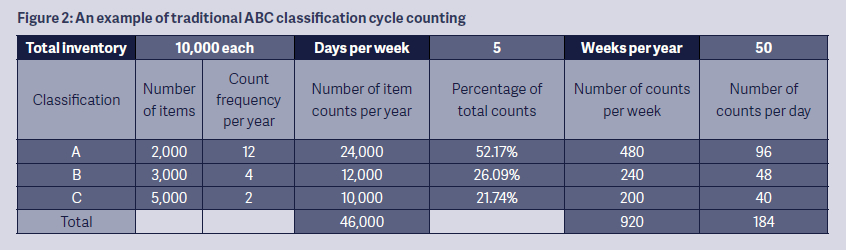The factor determining when an item class should be reviewed depends on the likelihood of an inventory discrepancy. In fact, it is the probability of item variance that should drive a cycle counting program. A static system is a serious problem if inventory accuracy declines. In such a case, the count interval should narrow and daily counts should increase so that items are counted as complete in a shorter timeframe. The opposite also is true: If inventory accuracy increases, the count interval will widen and the number of items counted will decline. Furthermore, the conventional model does not consider that each classification will have its own inventory accuracy target. Because A items will have a higher accuracy target than slow-moving C items, for example, they should be counted more often.

FREQUENCY AND QUANTITY

Now that the importance of item variance probability has been explained, the next critical issue becomes determining cycle count frequency and quantity. Frequency describes the interval of time separating the physical countings of the inventory of an item. Obviously, the more times an item’s inventory balance is reviewed and corrective action taken to eliminate the sources of variance, the greater the inventory accuracy. Of course, counting every item as much as possible is unmanageable—not to mention unnecessary. What, then, should be the review frequency for an effective cycle counting program? There are three possibilities:

1. Progress-based: In this model—which typically is used with zone and location-audit cycle counting methods—items in a set number of warehouse locations are counted daily. Often termed the “block method,” the counter literally takes a wall-to-wall inventory of all items in a set series of specified storage locations. The counter inventories everything found, whether or not the items are on the cycle count worksheet. The number of stocking locations counted each day then are reviewed within a predefined time span. For instance, a counter is expected to check all of the inventory located in 15 bays in the pallet rack area within 1 hour. When all of the locations within the warehouse have been counted, the process begins again with the first series of locations. The advantages of this method are that all items within the warehouse are given equal weight and that it does a very good job of finding inventory that is misplaced or no longer stocked. On the other hand, low-transaction items are treated the same way as high-transaction items, which means that the interval between the review cycle of the vital few in inventory is too long and that considerable time and energy are spent reviewing items with low probability of inventory variance.

2. Heuristic: The way to overcome the drawbacks of the progress-based method is to segregate the inventory into classes or locations based on the probability of variance. High-probability variance classes or locations are counted more frequently. The interval determining cycle count frequency is established by applying a reasonable heuristic. Figure 2 offers a simple example. A potential disadvantage to a heuristically determined interval is that it may be insensitive to the probability that the review interval will need to change as inventory accuracy improves.

3. Probability-based. The solution to the challenges associated with a heuristic approach is to use the same division of items into classes but then add a target inventory accuracy percent and the probability that a stock variance will occur within each class. These factors then are used in a formula to determine the review interval and the number of items to be counted. This formula enables the calculation of count intervals based on inventory accuracy targets by ABC class:

(1 – Accuracy Target) Accuracy Target × Variance Probability

Figure 3 is an illustration of an inventory of 10,000 items divided into three classes.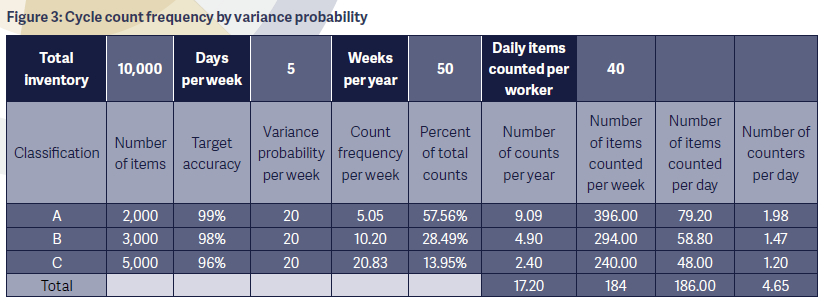The key to the spreadsheet is calculating the count frequency (portrayed by week) for each classification. The calculation is composed of several values, including

• total inventory (10,000)
• historical variance per class (20 for each of the three classes)
• number of items in each of the three classes (2,000, 3,000, and 5,000, respectively)
• target accuracy per class (99 percent, 98 percent, and 96 percent, respectively)
• number of work weeks per year (50)
• number of work days per week (5).

Let’s begin by determining the probability of error. Say the current accuracy for all three inventory classes is a respectable 90 percent. This means that about 10 percent × 10,000 items, all divided by 50 weeks, or a variance probability of 20, has been detected for each class each week.

Once the variance probability has been identified, it is possible to calculate the count frequency per week by applying the count frequency formula. A critical part of this calculation is identifying the target accuracy percentage linked to each classification. For example, determining the count frequency for class A items requires using the target accuracy of 99 percent in the formula as follows:This translates to a total of 9.9 complete cycles a year. Each cycle consists of a total of 396 items counted per week and 79 per day. If a counter can count an average of 40 items per day, this would mean that it would take about 2 counters to perform the count.

The most important aspect of this formula is that it is dynamic. For example, the central reason for cycle counting is determining the root cause of count variance and validating inventory accuracy controls. As the sources of variance are eliminated, the probability of variance should decrease accordingly. For instance, assume that inventory control is able to reduce the weekly variance of A items from 20 to 15. The results of the change would be as shown in Figure 4.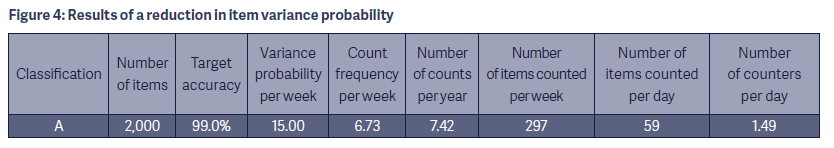The frequency to complete a count of all 2,000 A items goes from 5.05 to 6.73 weeks and from 9.90 counts per year to 7.42. In addition, the number of items counted per day decreases from 79 to 59, requiring 1.49 counters versus the former 1.98. As the probability of count variance moves toward 0 percent, both the need to perform a complete cycle count and the number of items counted each cycle are diminished.

Using probability in the development of the cycle count also exposes serious defects when compared with the heuristic method. The source of the gap is that, although cycle counting using probability is dynamic, the heuristic model is static. For example, if the cycle count program had succeeded in reducing the probability of class A item error from 20 to 15, then the gap between the dynamic cycle count and the static heuristic would grow. The reduction in probability would automatically adjust the cycle counting program, while it would be up to the planners to manually make the change. The gap between the two models is visible in Figure 5.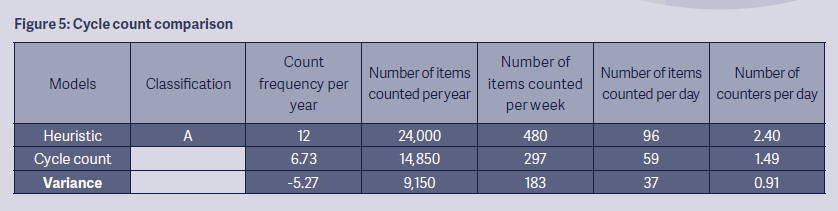In this situation, the inventory variance probability of A items has been reduced to 15, which results in about 7 cycle counts per year. When compared with the heuristic model, the probability model has cut the number of cycles by about 5 per year. In addition, the number of class A items counted for an entire year has declined by a little more than 9,000, with a difference of 183 counts per week and 37 counts per day. An important result is the reduction of 1 counter per day who could be freed up to perform other tasks.

PLAYING THE PROBABILITIES

Employing the variance probability formula to manage a cycle counting system offers numerous distinct advantages. First, the system is a direct reflection of the probability of inventory variance at the current time, rather than a static system. In addition, the application of the variance probability formula enables the continuous calculation of more meaningful cycle counting intervals and quantities based on the target inventory accuracies by product class.

An additional benefit is using individual count variances as they occur as the basis for root-cause review and variance elimination. The number of unsolved variances constitutes the variance probability used in the calculation of the next cycle count.

Yet another advantage is that the cost to perform the cycle count reflects the current inventory variance probabilities and eliminates unnecessary counting as found in a static system. The fact that the probability model enables management to not only see the costs, but also how many counters will be needed at any one given time, is a significant help in labor management.

Lastly, and perhaps most importantly, a probability-based cycle counting system enables inventory managers to focus their efforts on ensuring that a versatile inventory control tool will drive the ongoing accuracy of the business’s inventory assets.

CYCLE COUNTING BEYOND THE ABC’s

Although ABC classification is the most widespread method, cycle counts can be performed in many different ways. Following are brief descriptions of other techniques:

• In the zone method—which is particularly good for items with fixed locations—the count schedule starts with the first location in a zone and continues daily until the last location is reached. At this point, the count begins again at the first location.
• The location-audit method is best used when items are stored randomly. For this technique, a set number of locations is counted and their inventory counts are validated each day.
• When using the special-counts method, items are selected to be counted based on criteria such as negative or zero balances, shipment or receipt of items, fill shortages, and others.

David Ross, PhD, CFPIM, CSCP, is senior manager of professional development at APICS. He may be contacted at dross@apics.org.